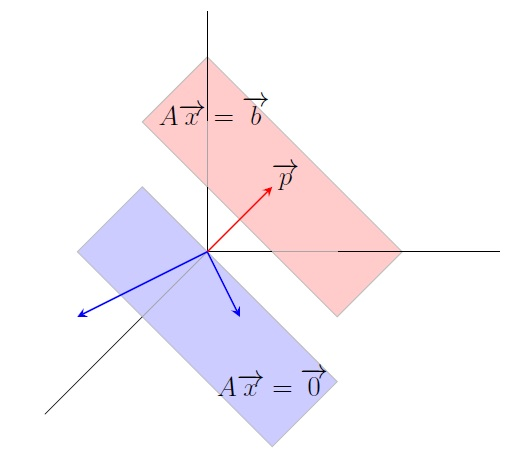Linear Algebra Home

## Geometry of Solution Sets

Homogeneous linear system: A system of linear equations is homogeneous if its matrix equation is $$A\overrightarrow{x}=\overrightarrow{0}$$. Note that $$\overrightarrow{0}$$ is always a solution called the trivial solution. Any nonzero solution is called a nontrivial solution.

Example.

1. $\begin{eqnarray*} \begin{array}{rcrcrcr} x_1&+&x_2 &-&x_3&=&0\\ &&3x_2&-&2x_3&=&0 \end{array} \end{eqnarray*}$ The corresponding matrix equation $$A\overrightarrow{x}=\overrightarrow{0}$$ has the solution set $\left\lbrace s \left[\begin{array}{r} 1\\ 2\\ 3 \end{array} \right] \; |\; s\in \mathbb R \right\rbrace$ which is also denoted by $$\operatorname{Span}\left\lbrace \left[\begin{array}{r} 1\\ 2\\ 3 \end{array} \right]\right\rbrace$$. This solution set corresponds to the points on the line in the 3-space $$\mathbb R^3$$ passing through the point $$(1,2,3)$$ and the origin $$(0,0,0)$$. Recall that the vector $$\left[\begin{array}{r} 1\\ 2\\ 3 \end{array} \right]$$ is the position vector of the point $$(1,2,3)$$ which is a directed line segment from the origin $$(0,0,0)$$ to the point $$(1,2,3)$$.

2. $x_1-x_2-2x_3=0$ The corresponding matrix equation $$A\overrightarrow{x}=\overrightarrow{0}$$ has the solution set $\left\lbrace s \left[\begin{array}{r}1\\ 1\\ 0 \end{array} \right] +t \left[\begin{array}{r}2\\ 0\\ 1 \end{array} \right] \; |\; s,t\in \mathbb R \right\rbrace =\operatorname{Span}\left\lbrace \left[\begin{array}{r} 1\\ 1\\0 \end{array} \right],\;\left[\begin{array}{r} 2\\0\\1 \end{array} \right] \right\rbrace.$ This solution set corresponds to the points on the plane in the 3-space $$\mathbb R^3$$ passing through the points $$(1,1,0)$$, $$(2,0,1)$$, and the origin $$(0,0,0)$$.

Remark. If $$A\overrightarrow{x}=\overrightarrow{0}$$ has $$k$$ free variables, then its solution set is the span of $$k$$ vectors.

The solution set of $$A\overrightarrow{x}=\overrightarrow{0}$$ is $$\operatorname{Span}\{\overrightarrow{v_1},\ldots,\overrightarrow{v_k}\}$$ for some vectors $$\overrightarrow{v_1},\ldots,\overrightarrow{v_k}$$.
The solution set of $$A\overrightarrow{x}=\overrightarrow{b}$$ is $$\{\overrightarrow{p}+\overrightarrow{v}\:|\: A\overrightarrow{v}=\overrightarrow{0}\}$$ where $$A\overrightarrow{p}=\overrightarrow{b}.$$
So a nonhomogenous solution is a sum of a particular solution and a homogeneous solution. To justify it, let $$\overrightarrow{y}$$ be a solution of $$A\overrightarrow{x}=\overrightarrow{b}$$, i.e., $$A\overrightarrow{y}=\overrightarrow{b}$$. Then $A(\overrightarrow{y}-\overrightarrow{p})=\overrightarrow{b}-\overrightarrow{b}=\overrightarrow{0}.$ Then $$(\overrightarrow{y}-\overrightarrow{p})=\overrightarrow{v}$$ where $$A\overrightarrow{v}=\overrightarrow{0}$$. Thus $$\overrightarrow{y}=\overrightarrow{p}+\overrightarrow{v}$$.
Geometrically we get the solution set of $$A\overrightarrow{x}=\overrightarrow{b}$$ by shifting the solution set of $$A\overrightarrow{x}=\overrightarrow{0}$$ to the point whose position vector is $$\overrightarrow{p}$$ along the vector $$\overrightarrow{p}$$.

Example. The nonhomogeneous system $$x_1-x_2-2x_3=-2$$ has a particular solution $$\overrightarrow{p}=\left[\begin{array}{r} 1\\ 1\\ 1 \end{array} \right]$$. The corresponding homogeneous system $$x_1-x_2-2x_3=0$$ has the solution set $\left\lbrace s \left[\begin{array}{r}1\\ 1\\ 0 \end{array} \right] +t \left[\begin{array}{r}2\\ 0\\ 1 \end{array} \right] \; |\; s,t\in \mathbb R \right\rbrace.$ Thus the solution set of the nonhomogeneous system $$x_1-x_2-2x_3=-2$$ is $\left\lbrace \left[\begin{array}{r} 1\\ 1\\ 1 \end{array} \right] +s \left[\begin{array}{r}1\\ 1\\ 0 \end{array} \right] +t \left[\begin{array}{r}2\\ 0\\ 1 \end{array} \right] \; |\; s,t\in \mathbb R \right\rbrace.$The solution set of $$A\overrightarrow{x}=\overrightarrow{b}$$ is a translation of that of $$A\overrightarrow{x}=\overrightarrow{0}$$

Last edited